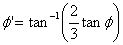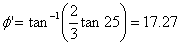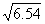HOMEWORK SOLUTION

HW #12

CE 353

GEOTECHNICAL ENGINEERING I

(Question no. 10.2, 10.4, 10.8, ..Al-Khafaji textbook)10.2     Square footing B = 1.5 m

D = 1.2 m

Soil                  sm = 17.6 kN/m3

c   = 50 kN/m2

f   = 30o

SF = 3

Condition         General shear failure

Question:          Allowable bearing capacity of footing

Solution:           for f = 30o  we obtain   Nc =33 ; Nq = 19.5 ; Ng = 17

For square footing

q ult      = 1.3 c Nc + q Nq + 0.4 g B Ng

= (1.3x50x33)+(19.5x1.2x22.5)+(0.4x17.6x1.5x17)

= 2145+526.5+179.52

= 2851 kN/m2

q all      = q ult/SF = 2851/3 = 950.3 kN/m2

Q all     = q all x B2 = 950.3 x 1.52

= 2138.27 kN

10.4     Strip footing      B = 5 ft

D = 4 ft

Soil                  sm = 100 pcf

c   = 750 pcf

f   = 25o

SF = 3

Condition         General shear failure

Question:          Allowable bearing capacity of footing

Solution:from fig 10.6 f=17.23 we obtain            Nc = 10 ; Nq = 3 ; Ng = 1.1

For strip footing:

q ult      = 2/3 c Nc’ + q Nq’ + 0.5 g B Ng'

= (2/3x750x10)+(100x4x3)+(0.5x100x5x1.1)

= 5000+1200+275

= 6475 psf

q all      = q ult/SF = 6475/3 = 2158.3 psf

10.8     Square footing Q all  = 1200 kN

D       = 1.5 m

Soil                  sm = 18 kN/m3

qu  = 120 kN/m2

f   = 0o

SF = 2.5

Condition         General shear failure

Question:          Dimension of footing

Solution:           for f = 0o  we obtain   Nc =5.53 ; Nq = 1.0 ; Ng = 0

q ult = (Q all x 2.5)/B2 = 3000/B2

For square footing

q ult                  = 1.3 c Nc + q Nq + 0.4 g B Ng

(3000/B2)         = (1.3x60x5.53)+(18x1.5x1)+(0)

= 458.34 kN/m2

B2           = 3000/458.34 =  6.54

B          == 2.558 ~ 2.6 m